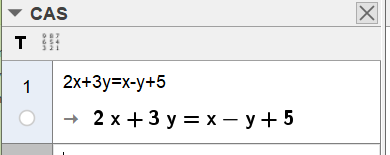# fixing an equation to input form

rsingkhuwal4445 shared this question 2 years ago

If I type 2x+3y=x-y+5 input bar, the output in algebra view is x+4y=5, then i change it to input form by right clicking the equation and selecting the input form from the option available. but everytime when i input new equation in input box linked to the equation, the output is always of the form ax+by=c. How can i fix the output always in the input form?1

you can type this kind of equations in CAS. CAS will keep this form and you can work with it further in CAS.1

thank you for the support but i have to use the equation as an object along with the text and wanted to make dynamic ggb file where i can change the equation using input box1